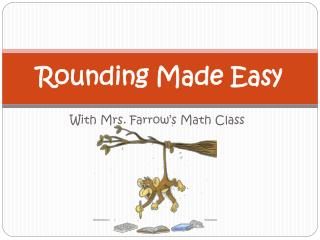DownloadDownload Presentation# Rounding Made Easy

Download Presentation## Rounding Made Easy

- - - - - - - - - - - - - - - - - - - - - - - - - - - E N D - - - - - - - - - - - - - - - - - - - - - - - - - - -
##### Presentation Transcript

1. Rounding Made Easy With Mrs. Farrow’s Math Class

2. Step 1: Identify the Place • Read the directions or word problem and identify the place you need to round to. EXAMPLE: Directions: Round to the nearest ten thousand. 286, 431

3. Step 2: Look to the RIGHT • Look at the number to the RIGHT of the place you are rounding to. EXAMPLE: 286,431

4. Step 3: 5 and or 4 and • Is the number to the right 5 and above or 4 and below? 0 1 2 3 4 5 6 7 8 9

5. Step 4: Round • Say to yourself: Five and above, give it a shove! Four and below, let it go! • If the number to the right is 5 or above you give the number you are rounding a shove up. • If the number to the right is 4 and below you leave the number you are rounding alone. EXAMPLES Round to the nearest ten thousand. • 286, 431 6 is bigger than 5 so we round the 8 up to a 9. 2. 472, 544 2 is less then 4 so you leave the 7 alone.

6. Step 5: Add the Zeroes • Change all numbers after the place you are rounding to zeroes. EXAMPLES Directions: Round to the nearest ten thousands. • 286, 431 = 290,000 2. 472,544 = 470,000

7. Let’s try one together! • Directions: Round 2, 726,311 to the nearest million. • What number is in the millions place? 2, 726,311 • What number is to the right? 2,726,311 • Is the number 5 and above, or 4 and below? 7 is greater than 5 so we bump the 2 up to a 3. • What is the final step? Change all of the numbers after the 2 to 0’s. • What is the final answer? 3,000,000 Spectacular!